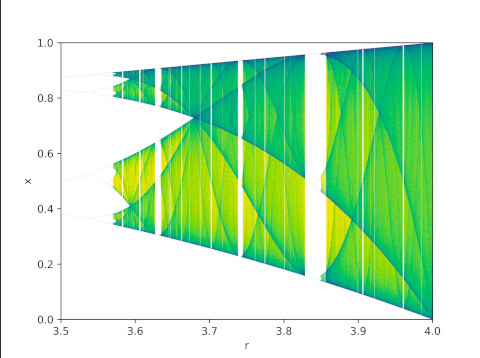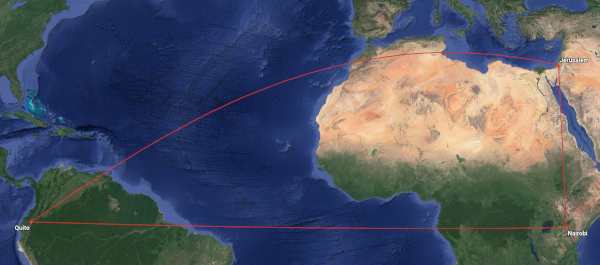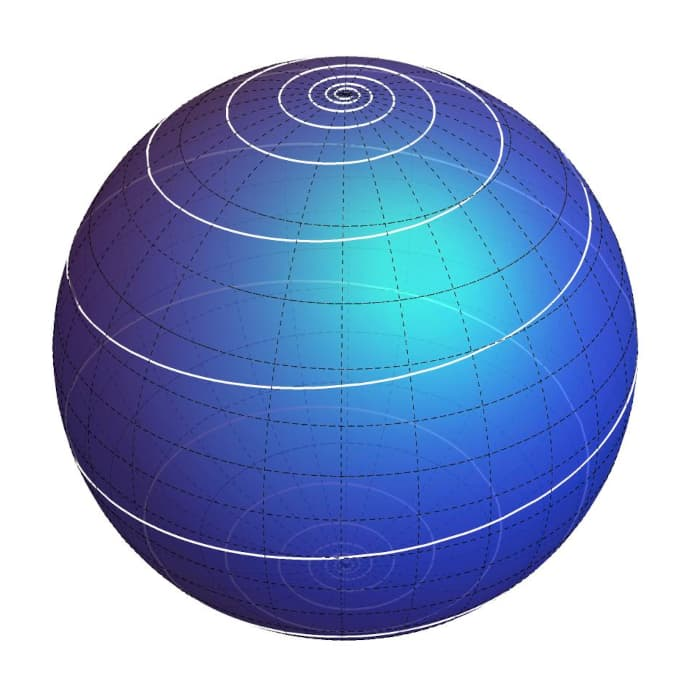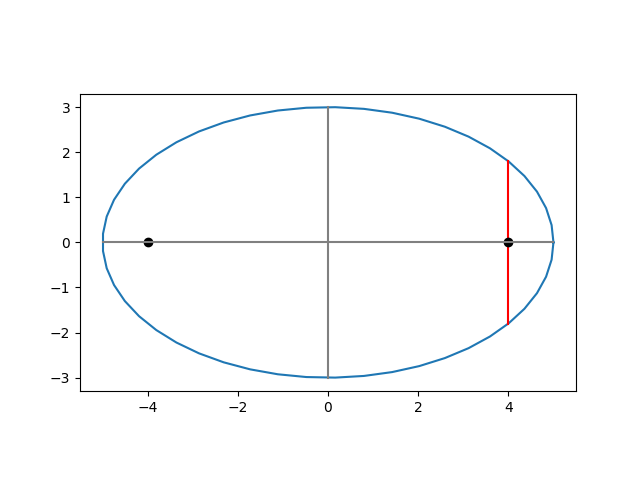# 222nd Carnival of Mathematics

A blog carnival is a round up of recent blog posts. The Carnival of Mathematics is a long-running carnival of blog posts on mathematical topics. This is the 222nd edition of the carnival.

By longstanding tradition, the nth Carnival of Mathematics must begin with trivia about the number n, and so here are five facts about the number 222.

• There are six groups of order 222, and 222 groups of order 912.
• 222=9+87+6×5×4+3+2+1.
• You can encode the number 222 in the Major mnemonic system as “unknown” or “one-on-one.”

## The posts

Gil Kalai looks at what happens when you ask ChatGPT to solve Elchanan Mossel’s dice problem.

Γιώργος Πλούσος (@plousos2505 on the social media platform formerly know as Twitter) posted an image showing how to compute π via a sequence of approximations requiring only basic geometry.

David Eppstein’s blog 11011110 has a post on Pyramidology. It’s particularly fitting to have a post from 1101110 in this carnival. As the author explains in his About page,

This journal’s name comes from interpreting my initials as the hexadecimal number 0xDE (decimal 222) and then converting the result to binary.

The blog ThatsMaths has a post on Sharkovsky numbering, Oleksandr Sharkovsky (1936–2022), and Sharkovsky’s Theorem. This post includes the beautiful image from Wikimedia.The post Patterns of reality by Timothy Williamson gives an overview of logic from basics to the work of Gödel and Turing.

Larissa Fedunik-Hofman posts an interview with Andrew Krause discussing dynamical system.

Finally, we have Matthew Scroggs’ Advent calendar of math puzzles.

To see previous editions of the carnival, or to submit a post to the next edition, see the Carnival of Mathematics page on Aperiodical.

# Wrapping Christmas lights around a tree trunk

Suppose you want to wrap Christmas lights around a tree trunk that we can approximate by a cylinder of radius r.

You want to wrap lights around the tree in a helix, going up a distance h every time you go around the tree once. What length of lights do you need to make n turns around the tree?

You can model the lights as a parametric curve with equation

(r cos t, r sin t, ht/2π)

If t ranges from 0 to T, the corresponding curve length is

(r² + h²/4π²)1/2 T

and you can set T =2πn if you want to find the length of n turns.

How much does h matter? Not much if h is less than r, as is often the case when wrapping a tree trunk with Christmas lights. My daughter Allison discovered this while wrapping lights around our pine tree, and then I wrote this post adding the math details.

If we expand (r² + h²/4π²)1/2 as a function of h in a Taylor series centered at 0 we get

(r² + h²/4π²)1/2  = r + / 8π²r + O(h4).

For example, suppose a tree is r = 10 inches in diameter and move h = 4 inches vertically with each turn. To complete one turn we let T = 2π. The exact length of one turn is

(r² + h²/4π²)1/2  T = (10² + 4²/4π²)1/2 (2π) = 62.96 inches.

If we ignore the h term we get

rT = 10 (2π) = 62.83 inches.

In short, the length of n turns around the tree is 2πrn, the same as 10 circles around the tree. The difference in length between a helix with n turns and n circles is negligible, provided h is smaller than r. Even if h = r = 10 in the example above, we’d get a length of 63.6 inches and our approximation would still be off by less than an inch per turn.

The heart of this calculation pops up frequently in various contexts. For example, the same calculation appears in the post It doesn’t matter much if the tape is straight.

# F# and G

I was looking at frequencies of pitches and saw something I hadn’t noticed before: F# and G have very nearly integer frequencies.

To back up a bit, we’re assuming the A above middle C has frequency 440 Hz. This is the most common convention now, but conventions have varied over time and place.

We’re assuming 12-tone equal temperament (12-TET), and so each semitone is a ratio of 21/12. So the nth note in the chromatic scale from A below middle C to A above middle C has frequency

220 × 2n/12.

I expected the pitch with frequency closest to integer would be an E because a perfect fifth above 220 Hz would be exactly 330 Hz. In equal temperament the frequency of the E above middle C is 329.6 Hz.

The frequency of F# is

220 × 29/12 Hz = 369.9944 Hz.

The difference between this frequency and 370 Hz is much less than the difference between equal temperament and other tuning systems.

The frequency of G is

220 × 25/6 Hz = 391.9954 Hz

which is even closer to being an integer.

In more mathematical terms, stripped of musical significance, we’ve discovered that

23/4 ≈ 37/22

and

25/6 ≈ 98/55.

# Straight on a map or straight on a globe?

Straight lines on a globe are not straight on a map, and straight lines on a map are not straight on a globe.

A straight line on a globe is an arc of a great circle, the shortest path between two points. When projected onto a map, a straight path looks curved. Here’s an image I made for a post back in August.The red lines form a spherical triangle with vertices at Quito, Nairobi, and Jerusalem. The leg from Quito to Nairobi is straight because it follows the equator. And the leg from Nairobi to Jerusalem is straight because it follows a meridian. But the leg from Quito to Jerusalem looks wrong.

If you were flying from Quito to Jerusalem and saw this flight plan, you might ask “Why aren’t we flying straight there, cutting across Africa rather than making a big arc around it? Are we trying to avoid flying over the Sahara?”

But the path from Quito to Jerusalem is straight, on a globe. It’s just not straight on the map. The map is not the territory.

Now let’s look at things from the opposite direction. What do straight lines on a map look like on a globe? By map I mean a Mercator projection. You could take a map and draw a straight line from Quito to Jerusalem, and it would cross every meridian at the same angle. A pilot could fly from Quito to Jerusalem along such a path without ever changing bearing. But the plane would have to turn continuously to stay on such a bearing, because this is not a straight line.

A straight line on a Mercator projection is a spiral on a globe, known as a loxodrome or a rhumb line. If a plane flew on a constant bearing from Quito but few over Jerusalem and kept going, it would spiral toward the North Pole. It would keep circling the earth, crossing the meridian through Jerusalem over and over, each time at a higher latitude. On a polar projection map, the plane’s course would be approximately a logarithmic spiral. The next post goes into this in more detail.I made the image above using the Mathematica code found here.

Although straight lines the globe are surprising on a map, straight lines on a map are even more surprising on a globe.

# Four views of multisets

This post will define multisets and basic operations on multisets.

We’ll view union, intersection, inclusion and sum each from four perspectives:

1. Examples with words
2. Example with prime factorization
3. Using Python’s  multiset module
4. Multisets as functions

# Latus rectum of an ellipse

Ellipses have been studied for over two thousand years, and so some of the terminology is ancient and sounds odd to modern ears. One such term is latus rectum. What is the latus rectum and does have it anything to do with anatomy?

This post defines the latus rectum for an ellipse. See the next post for a more general definition that extends to parabolas and hyperbolas.

## Background

An ellipse has two foci, two points such that the distance to one plus the distance to the other remains constant for every point on the ellipse. For a circle, the two foci coincide with the center.

Given an ellipse in the plane, we can pick a coordinate system so that our ellipse has equation

x²/a² + y²/b² = 1

where ab > 0. Here a is called the semi-major axis and b the semi-minor axis. The two foci are located at ±c where

c² = a² – b².

The ratio c/a is the eccentricity. As discussed here, eccentricity can be moderately large and yet the ellipse still be close to a circle.

## Definition

Pick one of the foci, say the one located at (c, 0). Then the line segment through the focus, parallel to the minor axis and connecting two points on the ellipse, is called the latus rectum. Its length is 2b² / a.

## Example

Let the semi-major axis be a = 5 and the semi-minor axis be b = 3. Then c = 4 and so the foci are located at (-4, 0) and (4, 0).

When x = 4, the equation of the ellipse tells us

16/25 + y²/9 = 1

and so y = ±9/5. So the latus rectum is the line connecting (4, -9/5) and (4, 9/5), the red vertical line below.## Why the name?

The first part latus mean side and the second part rectum means straight. According to Etymonline, rectum was

the name given to the lowest part of the large intestine by Galen, who so called it because he dissected only animals whose rectum (in contradistinction to that of man) is really straight.

So latus rectum means straight side. If you wanted to draw straight sides on an ellipse, where would you put them? Through the foci seems like as good a choice as any.

An interesting fact about the latus rectum is that its length is the diameter of a kissing circle tangent to the vertex of the ellipse. In other words, the semi-latus rectum, half the length of the latus rectum, is the radius of curvature at the vertex.

The dashed orange circle below has radius 9/5, equal to the semi-latus rectum. So the radius of curvature at the right end of the ellipse is 9/5 and the curvature is 5/9.# Branch cuts for elementary functions

As far as I know, all contemporary math libraries use the same branch cuts when extending elementary functions to the complex plane. It seems that the current conventions date back to Kahan’s paper . I imagine to some extent he codified existing practice, but he also settled some issues, particularly regarding floating point implementation.

I’ve verified that the following branch cuts are used by Mathematica, Common Lisp, and SciPy. If you know of any software that follows other conventions, please let me know in a comment.

The conventional branch cuts are as follows.

• sqrt: [-∞, 0)
• log: [-∞, 0]
• arcsin: [-∞, -1] and [1, ∞]
• arccos: [-∞, -1] and [1, ∞]
• arctan: [-∞i, –i] and [i, ∞i]
• arcsinh: [-∞i, –i] and [i, ∞i]
• arccosh: [-∞, 1]
• arctanh: [-∞, -1] and [1, ∞]

## Related posts

 W. Kahan. Branch Cuts for Complex Elementary Functions or Much Ado About Nothing’s Sign Bit. The State of the Art in Numerical Analysis. Clarendon Preess (1987).

# Literate programming to reduce errors

I had some errors in a recent blog post that might have been eliminated if I had programmatically generated the content of the post rather than writing it by hand.

I rewrote the example in this post in using org-mode. My org file looked like this:

```    #+begin_src python :session :exports none
lax_lat =   33.94
lax_lon = -118.41
iah_lat =   29.98
iah_lon =  -95.34
#+end_src

Suppose we want to find how far a plane would travel
between Los Angeles (LAX) and Houston (IAH),
...

#+begin_src python :session :exports none
a = round(90 - lax_lat, 2)
b = round(90 - iah_lat, 2)
#+end_src

/a/ = src_python[:session :results raw]{f"90° – {lax_lat}° = {a}°"}

and

/b/ = src_python[:session :results raw]{f"90° – {iah_lat}° = {b}°"}

...
```

Here are some observations about the experience.

First of all, writing the post in org-mode was more work than writing it directly, pasting in computed values by hand, but presumably less error-prone. It would also be easier to update. If, for example, I realized that I had the wrong coordinates for one of the airports, I could update the coordinates in one location and everything else would be updated when I regenerated the page.

I don’t think this was the best application of org-mode. It’s easier to use org-mode like a notebook, in which case you’re not trying to hide the fact that you’re mixing code and prose. I wanted to insert computed values into the text without calling attention to the fact that the values were computed. This is fine when you mostly have a text document and you only want to insert a few computed values. When you’re doing more computing it becomes tedious to repeatedly write

`    src_python[:session :results raw]{...}`

to insert values. It might have been easier in this case to simply write a Python program that printed out the HTML source of the example.

There are a couple advantages to org-mode that weren’t relevant here. One is that the same org-mode file can be exported to multiple formats: HTML, LaTeX, ODT, etc. Here, however, I was only interested in exporting to HTML.

Another advantage of org-mode is the ability to mix multiple programming languages. Here I was using Python for everything, but org-mode will let you mix dozens of languages. You could compute one result in R, another result in Haskell, pass both results as arguments into some Java code, etc. You could also include data tables and diagrams in your org-mode file with your prose and code.

## Literate programming

In general, keeping code and documentation together reduces errors. Literate programming may be more or less work, depending on the problem, but it reduces certain kinds of errors.

The example above is sorta bargain-basement literate programming. The code being developed was very simple, and not of interest beyond the article it was used in. Literate programming really shines when used to develop complex code, as in the book Physically Based Rendering. (Update: The third edition of this book is available online.)

When code requires a lot of explanation, literate programming can be very helpful. I did a project in psychoacoustics with literate programming a few years ago that would have been hard to do any other way. The project required a lot of reverse engineering and research. A line of code could easily require a couple paragraphs of explanation. Literate programming made the code development much easier because we could develop the documentation and code together, explaining things in the order most suited to the human reader, not to the compiler.

# Law of cosines on a sphere

The previous post looked at the analog of the Pythagorean theorem on a sphere. This post looks at the law of cosines on a sphere.

Yesterday we looked at triangles on a sphere with sides a and b meeting at a right angle and hypotenuse c. Denote the angle opposite a side with the capital version of the same letter, so C is the angle opposite c. We assumed C is a right angle, but now we will remove that assumption.

The spherical analog of the law of cosines says

cos(c) = cos(a) cos(b) + sin(a) sin(b) cos(C).

Note that we have two kinds of angles: the arcs that make up the sides, and the angle formed by the intersection of arcs . So the cos(C) term at the end is different animal from the other terms in the equation.

If C is a right angle, cos(C) = 0 and the second term drops out, leaving us with the spherical counterpart of the Pythagorean theorem. But we do not require that C be a right angle.

## Application to air distance

Suppose we want to find how far a plane would travel between Los Angeles (LAX) and Houston (IAH), assuming it takes a great circle path. The lat/long coordinates of the two airports are (33.94°, -118.41°) and (29.98°, -95.34°).

Los Angeles and Houston are approximately at the same latitude, but even if they were at exactly the same latitude we couldn’t just find the flight distance by finding the length of the arc of constant latitude between them because that arc would not be part of a great circle.

To find the distance between LAX and IAH we form a triangle with vertices at LAX, IAH, and the north pole. Call the arc from LAX to the north pole a and the arc from IAH to the north pole b. Since latitude is the angle up from the equator, the angle down from the pole is 90° minus latitude. So

a = 90° – 33.94° = 56.06°

and

b = 90° – 29.98° = 60.02°

The arcs a and b meet at the angle C equal to the differences in the two longitudes. That is,

C = 118.41° – 95.34° = 23.07°.

The law of cosines

cos(c) = cos(a) cos(b) + sin(a) sin(b) cos(C)

reduces to

cos(c) = cos(56.06°) cos(60.02°) + sin(56.06°) sin(60.02°) cos(23.07°)

in our problem.

This tells us

cos(c) = 0.9402

from which we find c = 0.3477 radians or 19.92°. Assume the earth is a perfect sphere of radius r = 3959 miles. The arc length we’re after is r times c in radians, or 1376 miles.

## Related posts

 In the language of differential geometry, the arcs are geodesics on the sphere and the angles of intersection are measured in the tangent space at the intersection point.

# Naming probability functions

Given a random variable X, you often want to compute the probability that X will take on a value less than x or greater than x. Define the functions

FX(x) = Prob(Xx)

and

GX(x) = Prob(X > x)

What do you call F and G? I tend to call them the CDF (cumulative distribution function) and CCDF (complementary cumulative distribution function) but conventions vary.

The names of software functions to compute these two functions can be confusing. For example, Python (SciPy) uses the names `cdf` and `sf` (the latter for “survival function”) while the R functions to compute the CDF take an optional argument to return the CCDF instead .

In the Emacs calculator, the function `ltpn` computes the CDF. At first glace I thought this was horribly cryptic. It’s actually a very nice naming convention; it just wasn’t what I was expecting.

The “ltp” stands for lower tail probability and “n” stands for normal. The complementary probability function is `utpn` where “utp” stands for upper tail probability. Unlike other software libraries, Emacs gives symmetric names to these two symmetrically related functions.

“Lower tail” probability is clearer than “cumulative” probability because it leaves no doubt whether you’re accumulating from the left or the right.

You can replace the “n” at the end of `ltpn` and `utpn` with the first letters of binomial, chi-square, t, F, and Poisson to get the corresponding functions for these distributions. For example, `utpt` gives the upper tail probability for the Student t distribution .

The Emacs calculator can be quirky, but props to the developers for choosing good names for the probability functions.

## Related posts

 Incidentally, the CCDF cannot always be computed by simply computing the CDF first and subtracting the value from 1. In theory this is possible, but not in floating point practice. See the discussion of erf and erfc in this post for an explanation.

 These names are very short and only a handful of distribution families are supported. But that’s fine in context. The reason to use the Emacs calculator is to do a quick calculation without having to leave Emacs, not to develop production quality statistical software.